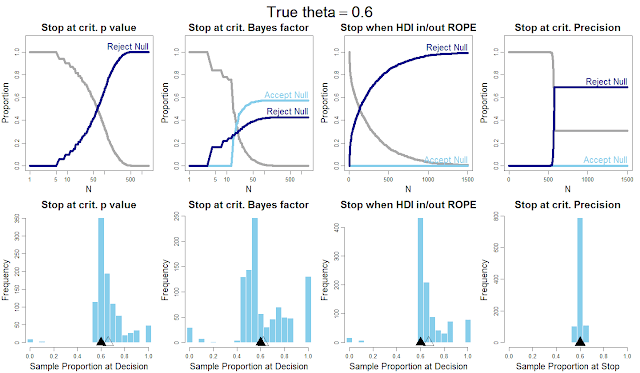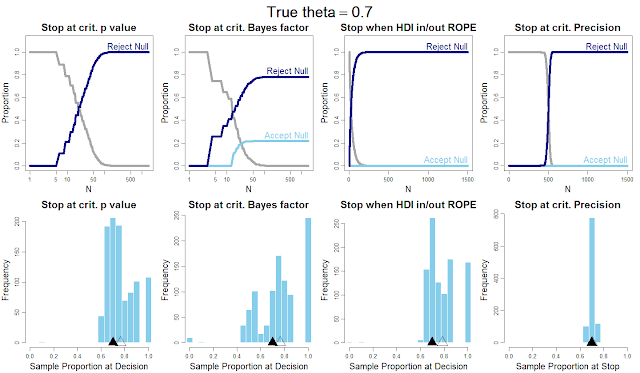Monday, November 4, 2013

Optional stopping in data collection: p values, Bayes factors, credible intervals, precision

This post argues that data collection should stop when a desired degree of precision is achieved (as measured by a Bayesian credible interval), not when a critical p value is achieved, not when a critical Bayes factor is achieved, and not even when a Bayesian highest density interval (HDI) excludes a region of practical equivalence (ROPE) to the null value.

Update: For expanded follow-up talk, from March 14, 2014 at U.C. Irvine, see:

It is a well-known fact of null-hypothesis significance testing (NHST) that when there is "optional stopping" of data collection with testing at every new datum (a procedure also called "sequential testing" or "data peeking"), then the null hypothesis will eventually be rejected even when it is true. With enough random sampling from the null hypothesis, eventually there will be some accidental coincidence of outlying values so that p < .05 (conditionalizing on the current sample size). Anscombe (1954) called this phenomenon, "sampling to reach a foregone conclusion."

Bayesian methods do not suffer from this problem, at least not to the same extent. Using either Bayesian HDI with ROPE, or a Bayes factor, the false alarm rate asymptotes at a level far less than 100% (e.g., 20-25%). In other words, using Bayesian methods, the null hypothesis is accepted when it is true, even with sequential testing of every datum, perhaps 75-80% of the time.

But not all is well with the Bayesian methods.Within the two Bayesian methods, the Bayes-factor method is far too eager to accept the null when it is not true. And both Bayesian methods, like the p-value method, give biased estimates of the parameter value when the null is not true, because they stop when extreme values are (randomly) obtained.

The proposed solution to the problem of biased estimates is to stop when a desired degree of precision is achieved, regardless of what it implies about the null hypothesis. This is standard procedure in political polling, in which sampling is designed to achieve a desired confidence bound on the estimate (e.g., "plus or minus 3 percentage points"), not to argue for one extreme or the other. I previously mentioned this proposal in a video (at 6:45 minutes) and alluded to it in an article (pp. 579-580) and in the book (Doing Bayesian Data Analysis; goals for power analysis, e.g., pp. 320-321), but surely I am not the first to suggest this; please let me know of precursors.

What follows is a series of examples of sequential testing of coin flips, using four different stopping criteria. The underlying bias of the coin is denoted θ (theta). Here are the four stopping rules:
• NHST: For every new flip of the coin, stop and reject the null hypothesis, that θ=0.50, if p < .05 (two-tailed, conditionalizing on the current N), otherwise flip again.
• Bayes factor (BF): For every flip of the coin, conduct a Bayesian model comparison of the null hypothesis that θ=0.50 against the alternative hypothesis that there is a uniform prior on θ. If BF > 3, accept null and stop. If BF < 1/3 reject null and stop. Otherwise flip again.
• Bayesian HDI with ROPE: For every flip of the coin, compute the 95% HDI on θ. If the HDI is completely contained in a ROPE from 0.45 to 0.55, stop and accept the null. If the HDI falls completely outside the ROPE stop and reject the null. Otherwise flip again.
• Precision: For every flip of the coin, compute the 95% HDI on θ. If its width is less than 0.08 (.8*width of ROPE) then stop, otherwise flip again. Once stopped, check whether null can be  accepted or rejected according to HDI with ROPE criteria.
The diagrams below show the results for when the null hypothesis is true, and for three cases in which the null hypothesis is not true. The panels show the results for Monte Carlo simulation of flipping (or spinning) a coin that has bias θ. In each case, there were 1,000 simulated experiments, and for each experiment the sample size was allowed to grow up to 1,500 flips. The upper panels in each figure show the proportion of the 1,000 experiments that decided to accept the null, reject the null, or remain undecided, as a function of the sample size. The light blue curve shows the proportion of decisions to accept the null, the dark blue curve shows the proportion of decisions to reject the null, and the grey curve shows the remaining undecided proportion.
The lower panels in each figure show a histogram of the 1,000 sample proportions of heads when the sequence was stopped. The solid triangle marks the true underlying theta value, and the outline triangle marks the mean of the 1,000 sample proportions when the sequence was stopped. If the sample proportion at stopping is unbiased, then the outline triangle would be superimposed on the solid triangle.Figure 1

Figure 1, above, shows the case of θ=0.50, that is, when the null hypothesis is true. The left column shows the behavior of the p-value stopping rule. As the sample size increases (notice that N is plotted on a logarithmic scale), the proportion of sequences that have rejected the null continues to rise -- in fact linearly with the logarithm of N. The lower left panel shows the distribution of sample proportions when the sequence stopped, that is, when the sequence had successfully rejected the null.

The second column of Figure 1 shows the results from using the Bayes-factor (BF) stopping rule. When the null hypothesis is true, it shows similar behavior to the HDI-with-ROPE stopping rule, but with smaller sample sizes. In fact, it can make decisions with very small sample sizes that yield very uncertain estimates of theta.

The third column of Figure 1 shows the results from using the HDI-with-ROPE stopping rule. You can see that there is some false rejection of the null for small sample sizes, but the false alarms soon asymptote. When the sample size gets big enough so the HDI is narrow enough to fit in the ROPE, the remaining sequences all eventually accept the null.

The fourth column of Figure 1 shows the results of using the precision stopping rule. Because the desired precision is a width of 0.08, a fairly large sample is needed. Once the desired HDI width is attained, it is compared with the ROPE to make a decision. The curves extend (misleadingly) farther right over larger N even though data collection has stopped.Figure 2

Figure 2 shows the results when the true bias is θ=0.60 (not 0.50). The left column shows that decision by p-value always rejects the null, but sooner that when θ=0.50. The second column shows that the BF still accepts the null hypothesis more than 50% of the time! The third column shows that the HDI-with-ROPE method always gets the right answer (in terms of rejecting the null) but can take a lot of data for the HDI to exclude the ROPE. The real news is in the lower row of Figure 2, which shows that the sample proportion, at the point of decision, over estimates the true value of θ, for all methods except stopping at desired precision. (Actually, there is a slight bias even in the latter case because of ceiling and floor effects for this type of parameter; but it's very slight.)Figure 3

The same remarks apply when θ=0.65 as in Figure 3. Notice that the BF still accepts the null almost 40% of the time! Only the stop-at-critical-precision method does not overestimate θ.Figure 4
The same remarks apply when θ=0.70 as in Figure 4. Notice that the BF still accepts the null about 20% of the time! Only the stop-at-critical-precision method does not overestimate θ.

Discussion:

"Gosh, I see that the stop-at-critical-precision method does not bias the estimate of the parameter, which is nice, but it can take a ton of data to achieve the desired precision. Yuck!" Oh well, that is an inconvenient truth about noisy data -- it can take a lot of data to cancel out the noise. If you want to collect less data, then reduce the noise in your measurement process.

"Can't the method be gamed? Suppose the data collector actually collects until the HDI excludes the ROPE, notes the precision at that point, and then claims that the data were collected until the precision reached that level. (Or, the data collector rounds down a little to a plausibly pre-planned HDI width and collects a few more data values until reaching that width, repeating until the HDI still excludes the ROPE.)" Yup, that's true. But the slipperiness of the sampling intention is the fundamental complaint against all frequentist p values, which change when the sampling or testing intentions change. The proportions being plotted by the curves in these figures are p values -- just p values for different stopping intentions.

"Can't I measure precision with a frequentist confidence interval instead of a Bayesian posterior HDI?" No, because a frequentist confidence interval depends on the stopping and testing intention. Change, say, the testing intention --e.g., there's a second coin you're testing-- and the confidence interval changes. But not the Bayesian HDI.

The key point: If the sampling procedure, such as the stopping rule, biases the data in the sample, then the estimation can be biased, whether it's Bayesian estimation or not. A stopping rule based on getting extreme values will automatically bias the sample toward extreme estimates, because once some extreme values show up by chance, sampling stops. A stopping rule based on precision will not bias the sample unless the measure of precision depends on the value of the parameter (which actually is the case here, just not very noticeably for parameter values that aren't very extreme).